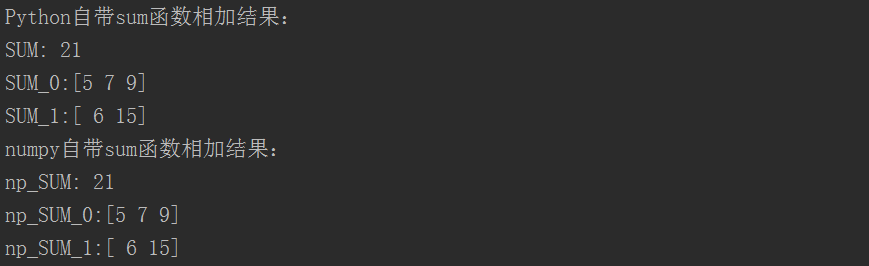• select sum(case when column&gt; 0 then column else 0 end) as u0sum(case when column &lt;0 then column else 0 end ) as d0 from tablename
select sum(case when column> 0 then column else 0 end) as u0,
sum(case when column <0 then column else 0 end ) as d0 from tablename 


展开全文mysql 负数
• Numpy中sum函数（Python自带sum函数）的作用是对元素求和。 无参时，所有全加； axis=0，按列相加； axis=1，按行相加； 下边通过例子来说明其用法： #!/usr/bin/env python # -*- coding:utf-8 -*- from numpy...
Numpy中sum函数（Python自带sum函数）的作用是对元素求和。

无参时，所有全加；
axis=0，按列相加；
axis=1，按行相加；
下边通过例子来说明其用法：

#!/usr/bin/env python
# -*- coding:utf-8 -*-
from numpy import *
import numpy as np

a = array([[1, 2, 3], [4, 5, 6]])
# Python自带sum函数相加
SUM = sum(a)  # 所有元素求和
SUM_0 = sum(a, 0)  # 按照列求和
SUM_1 = sum(a, 1)  # 按照行求和
print("SUM:"+str(SUM))
print("SUM_0:"+str(SUM_0))
print("SUM_1:"+str(SUM_1))

# numpy中sum函数相加
np_SUM = np.sum(a)  # 所有元素求和
print("np_SUM:"+str(np_SUM))
np_SUM_0 = np.sum(a, axis=0)  # 按照列求和
print("np_SUM_0:"+str(np_SUM_0))
np_SUM_1 = np.sum(a, axis=1)  # 按照行求和
print("np_SUM_1:"+str(np_SUM_1))

运行结果：展开全文numpy Python
•link
• ## python求和函数sum()详解

万次阅读 多人点赞 2019-04-06 21:32:44
python求和函数sum()详解 今天在学习的过程中，误用sum()函数，我又去查了查python sum()函数才恍然大悟。 我本来想算几个Int值相加的和，本以为很简单的事情，结果却很悲伤，例： >>>sum = sum(1,2,3) #...
python求和函数sum()详解
今天在学习的过程中，误用sum()函数，我又去查了查python sum()函数才恍然大悟。
我本来想算几个Int值相加的和，本以为很简单的事情，结果却很悲伤，例：
>>>sum = sum(1,2,3)         #结果很明显出现问题报错
TypeError: sum expected at most 2 arguments, got 3

傻乎乎的我以为只能算前两个数的和等于3，就又试了一下
>>>sum = sum(1,2)           #结果还是报错
TypeError: 'int' object is not iterable

其实，我们所了解sum()函数语法是这样的
sum(iterable[, start])
其中

iterable – 可迭代对象，如：列表(list)、元组(tuple)、集合(set)、字典(dictionary)。

start – 指定相加的参数，如果没有设置这个值，默认为0。

也就是说sum()最后求得的值 = 可迭代对象里面的数加起来的总和(字典:key值相加) + start的值(如果没写start的值，则默认为0) 所以，我所求的几个int值的和可以写成这样

>>>sum = sum([1,2,3])             # in list
6

如果我们加上start的话，应该是这样的
>>> sum = sum([1,2,3],5)          #in list +start
11
>>> sum = sum((1,2,3))            #in tuple
6
>>> sum = sum({1,2,3})            #in set
6
>>> sum = sum({1:5,2:6,3:7})      #in dictionary key
6
>>> sum = sum(range(1,4))         #in range()
6

学习这些过后，我们就可以正确的运用sum()函数了。
别指望着看一遍就能记住和整掌握什么 – 请看第二遍，第三遍。


展开全文python python求和
• ## python中sum函数

千次阅读 2017-01-25 17:13:50
python自带的sum函数（或者Numpy中的sum函数），无参时，所有全加；axis=0，按列相加；axis=1，按行相加 import numpy as np #python中自带的sum print(sum([[1,2,3],[4,5,5]])) print(sum([[1,2,3],[4,5,5]],...
版本：python3
python自带的sum函数（或者Numpy中的sum函数），无参时，所有全加；axis=0，按列相加；axis=1，按行相加
import numpy as np
#python中自带的sum
print(sum([[1,2,3],[4,5,5]]))
print(sum([[1,2,3],[4,5,5]],axis=0))
print(sum([[1,2,3],[4,5,5]],axis=1))
#Numpy中的sum
a = np.sum([[1,2,3], [4,5,5]]) #无参
print(a)
print(a.shape)
a = np.sum([[1,2,3], [4,5,5]],axis=0) #axis=0, 按列相加
print(a)
print(a.shape)
a = np.sum([[1,2,3], [4,5,5]],axis=1) #axis=1, 按行相加
print(a)
print(a.shape)
20
[5 7 8]
[ 6 14]
20
()
[5 7 8]
(3,)
[ 6 14]
(2,)

展开全文python numpy
• ## matlab中sum函数的用法

万次阅读 多人点赞 2019-06-21 16:47:26
参考：https://jingyan.baidu.com/article/6b97984db545971ca2b0bf98.html b=sum(a,dim); a表示矩阵； dim等于1或者2. 1表示每一列进行求和，2表示每一行进行求和； 表示每列求和还是每行求和； b表示求得的...
• sum函数主要为了矩阵的行、或者列的和。其中用axis这个参数来指定对行还是列求和，当没有指定axis参数的时候，就会对矩阵所有元素求和。 import numpy as np #生成一个2维矩阵 a = range(16) a = np.array(a) a =...
• sum函数用于矩阵列方向元素或向量的和，调用格式如下。 （1）B=sum(A)：若A向量，则返回所有元素的和；如A矩阵，则分别对其各列所有元素求和并返回结果。 （2）B=sum(A,dim)：分别对矩阵A中第dim维的所有...
• 在SQL语句中使用集合函数count()，返回结果肯定非负整数，这也是用来判断数据有无的一个很有效的方法； 然后在用sum()进行集合相加的时候，如果sum()的对象没有记录，那么不会返回0，而返回null，这么做，不会...数据库 mysql
• ## python中sum函数详解

千次阅读 2019-09-11 14:48:38
python内置的sum函数 对象是列表，元组，集合，可以混合起来使用 >>>sum([1,2,3],2) 8 >>>sum((1,2,3)) 6 >>>sum({1,2,3}) 6 >>>sum(range(5)) 10 numpy中sum...python
• 在使用matlab进行仿真时，出现使用sum函数报错，报错提示：【下标索引必须正整数类型或逻辑类型】，错误如下： 解决方法： 仔细检查程序运行区域是否出现自定义变量名称【sum】，如果有修改名称或删除后...matlab
• ## matlab中的sum函数

千次阅读 2018-05-12 16:28:56
sum函数用法：a = sum(x); %列求和，生成行向量a = sum(x, 1); %列求和，生成行向量a = sum(x, 2); %行求和，生成列向量a = sum(x(:)); %矩阵内所有元素求和，生成一个值&gt;&gt; x = [1,2,3;4,5,6;7,8,9] ...matlab
• Hibernate（分组查询等续）  使用聚集函数 ...Sum：求和函数 Avg：平均数函数  Count：统计函数 Session session = HibernateSessionFactory.getSession(); Transaction tx = ses
• ## python中的sum函数.sum(axis=1)

万次阅读 多人点赞 2013-11-29 16:02:29
看起来挺简单的样子，但是在给sum函数中加入参数。sum（a，axis=0）或者是.sum(axis=1) 就有点不解了 在我实验以后发现 我们平时用的sum应该是默认的axis=0 就是普通的相加  而当加入axis=1以后就是将一个矩阵...python numpy
• max,min,sum函数的使用 QueryWrapper<MemberLevel> queryWrapper = new QueryWrapper<>(); queryWrapper.select("max(level_sort) as levelSort"); 相当于sql : select max(level_sort) as levelSort...maxoracle select
• ## python中的sum函数

千次阅读 2016-11-02 17:15:46
sum(axis=0)是将一个矩阵的每一列向量相加 对一维数组，只有第0轴，没有第1轴 例如： import numpy as np np.sum([[0,1,2],[2,1,3],axis=1) 结果就是：array（[3,6]） c = np.array([[0, 2,...python
• ## matlab中sum函数求和

千次阅读 2015-05-21 17:41:25
matlab中sum函数求和 sum(x,2)表示矩阵x的横向相加，每行的和，结果是列向量。 而缺省的sum(x)就是竖向相加，每列的和，结果是行向量。
• 郑重声明，我写的是一个sum函数，虽然不知道python内置的是怎么实现的，在写之前，也从未看过源码 def sum(*args): return reduce(lambda x,y:x+y,args) 传的是可变参数，当然要是传其他的也好改 我自己感觉这...
• ## matlab中的sum函数的用法

万次阅读 多人点赞 2015-09-25 13:20:38
Matlab中的sum函数功能非常强大，但是如何使用不慎就会造成难以发现的错误。比如 A1 = [1, 3, 2] S = sum(A1) S_2 = sum(A1,2) S_1 = sum(A1,1) 输出结果： A1 =  1 3 2 S =  6 S_2 =  6 ...
• 1.tile函数： tile函数是模板numpy.lib.shape_base中的函数函数的形式是tile(A,reps) A的类型几乎所有类型都可以：array, list, tuple, dict, matrix以及基本数据类型int, string, float以及bool类型。 reps...python numpy shape
• ## Numpy之sum函数用法

万次阅读 2017-08-09 16:59:17
文档原文在这： https://docs.scipy.org/doc/numpy-1.10.1/reference/generated/numpy.sum.htmlnumpy.sum用法如下：numpy.sum(a, axis=None, ... 一个数组中给定轴上的元素的总和。 参数如下：a：array_like 类型numpy
• 在mysql中sum函数查出的最终结果为0的时候会显示null，这时候就需要将null转为0：方法1：使用IFNULL(expr1,expr2)函数： SELECT  IFNULL(sum(SS), 0.0) AS sum FROM  fd_order fo2 WHERE  fo2.BUYER_ID = '11' ...
• Python内置的sum函数 笔者的理解 拓展：NumPy中sum函数 相似度时看到的，碰到一个疑问的地方，先写下来。 《机器学习基础：相似度和距离度量究竟是什么》 Python内置的sum函数 作用：对可迭代对象进行求和...Python
• matlab中的 MAX函数的几种形式 　(1)max(a) 　(2)max(a,b) 　(3)max(a,[],dim) 　(4)[C,I]=max(a) 　(5)[C,I]=max(a,[],dim) 　max函数的结果 　(1)max(a) 　如果 a 是一个矩阵，比如 a=[1,2,3;4,5,6]，max(a)的意思...
• matlab中使用sum和cumsum两个加和函数，区别联系 参考matlab中cumsum函数详解 matlab中cumsum函数通常用于计算一个数组各行的累加值。在matlab的命令窗口中输入doc cumsum或者help cumsum即可获得该函数的帮助...
• ## python sum函数的用法

千次阅读 2018-12-27 10:40:20
需要用到python sum（）函数，总结了一下，不对之处烦请指出，谢谢。 1维情况下  （1）输入axis=0 import numpy as np a=np.array([1,2,3,4,5]) b=a.sum(axis=0) print(b)  输出结果：15, 也就是一维数组...
• 分析：我们可以用oracle的分析函数sum()来解决这个问题，通过换钱的时间升序，然后用总的金额减去前面总共还了多少，得到每次还了还欠的钱。With tmp As ( Select to_date('20140228','yyyymmdd') a,1000 b
• MySQL的SUM函数是用来找出记录中各种的字段的总和。要了解SUM函数考虑EMPLOYEE_TBL表具有以下记录：mysql> SELECT * FROM employee_tbl; +——+——+————+——————–+ | id | name | work_date | daily_...mysql...